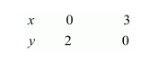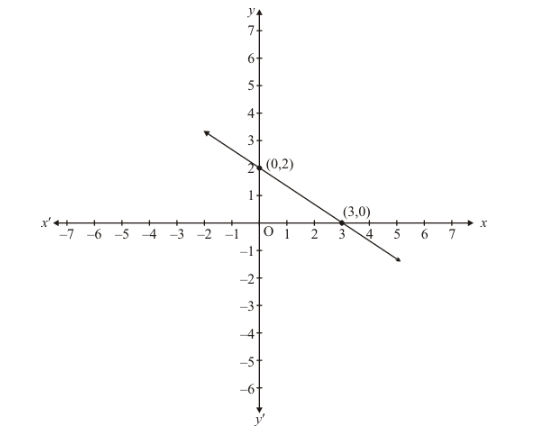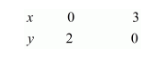# Show graphically that each one of the following systems of equations has infinitely many solutions:`
Question:

Show graphically that each one of the following systems of equations has infinitely many solutions:

$2 x+3 y=6$

$4 x+6 y=12$

Solution:

The given equations are

$2 x+3 y=6$ (i)

$4 x+6 y=12$ (ii)

Putting $x=0$ in equation $(i)$, we get:

$\Rightarrow 2 \times 0+3 y=6$

$\Rightarrow y=2$

$x=0, \quad y=2$

Putting $y=0$ in equation $(i,$, we get:

$\Rightarrow 2 x+3 x=6$

$\Rightarrow x=3$

$x=3, \quad y=0$

Use the following table to draw the graph.Draw the graph by plotting the two points $A(0,2)$ and $B(3,0)$ from table.Graph of the equation....(ii):

$4 x+6 y=12$. .(ii)

Putting $x=0$ in equation (ii) we get:

$\Rightarrow 4 \times 0+6 y=12$

$\Rightarrow y=2$

$x=0, \quad y=2$

Putting $y=0$ in equation $(i i)$, we get:

$\Rightarrow 4 x+6 \times 0=12$

$\Rightarrow x=3$

$x=3, \quad y=0$

Use the following table to draw the graph.Draw the graph by plotting the two points $C(0,2), D(3,0)$ from table.

Thus the graph of the two equations coincide

Consequently, every solution of one equation is a solution of the other.

Hence the equations have infinitely many solutions.